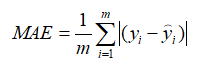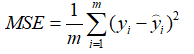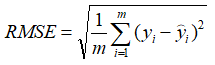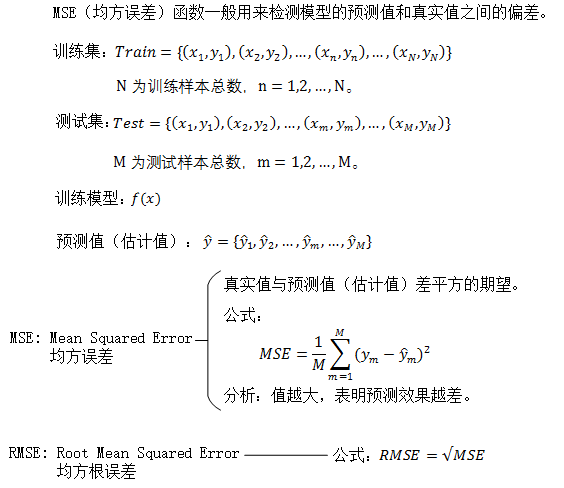• MAE、MAPE、MSE和RMSE的MATLAB代码
2022-04-18 15:44:39

平均绝对误差（MAE）

mae = mean(abs(YReal - YPred));

平均绝对百分比误差（MAPE）

mape = mean(abs((YReal - YPred)./YReal));

均方误差（MSE）

mse = (sum((YReal - YPred).^2)) ./ n;

均方根误差（RMSE）

rmse = sqrt(mean((YPred-YReal).^2));matlab
更多相关内容
• 回归算法中常用的评价指标：MAE、MSE和RMSE 1.MAE（Mean Absolute Error：平均绝对误差） 其中，表示真实值，表示预测值 2.MSE（Mean Square Error:均方误差） 3.RMSE（Root Mean Square Error:均方根误差...

回归算法中常用的评价指标：MAE、MSE和RMSE

1.MAE（Mean Absolute Error：平均绝对误差）其中，表示真实值，表示预测值

2.MSE（Mean Square Error:均方误差）3.RMSE（Root Mean Square Error:均方根误差）展开全文回归
• 今天小编就为大家分享一篇python之MSE、MAE、RMSE的使用，具有很好的参考价值，希望对大家有所帮助。一起跟随小编过来看看吧
• 前言分类问题的评价指标是准确率，那么回归算法的评价指标就是MSERMSE，MAE、R-Squared。下面一一介绍均方误差(MSE)MSE (Mean Squared Error)叫做均方误差。看公式image.png这里的y是测试集上的。用 真实值-预测值...

前言

分类问题的评价指标是准确率，那么回归算法的评价指标就是MSE，RMSE，MAE、R-Squared。下面一一介绍

均方误差(MSE)

MSE (Mean Squared Error)叫做均方误差。看公式image.png

这里的y是测试集上的。

用 真实值-预测值 然后平方之后求和平均。

猛着看一下这个公式是不是觉得眼熟，这不就是线性回归的损失函数嘛！！！ 对，在线性回归的时候我们的目的就是让这个损失函数最小。那么模型做出来了，我们把损失函数丢到测试集上去看看损失值不就好了嘛。简单直观暴力！

均方根误差(RMSE)

RMSE(Root Mean Squard Error)均方根误差。image.png

这不就是MSE开个根号么。有意义么？其实实质是一样的。只不过用于数据更好的描述。

例如：要做房价预测，每平方是万元(真贵)，我们预测结果也是万元。那么差值的平方单位应该是 千万级别的。那我们不太好描述自己做的模型效果。怎么说呢？我们的模型误差是 多少千万？。。。。。。于是干脆就开个根号就好了。我们误差的结果就跟我们数据是一个级别的可，在描述模型的时候就说，我们模型的误差是多少万元。

MAE

MAE(平均绝对误差)image.png

不用解释了吧。

R Squared

上面的几种衡量标准针对不同的模型会有不同的值。比如说预测房价 那么误差单位就是万元。数子可能是3，4，5之类的。那么预测身高就可能是0.1，0.6之类的。没有什么可读性，到底多少才算好呢？不知道，那要根据模型的应用场景来。

看看分类算法的衡量标准就是正确率，而正确率又在0～1之间，最高百分之百。最低0。很直观，而且不同模型一样的。那么线性回归有没有这样的衡量标准呢？答案是有的。

那就是R Squared也就R方image.png

光看这些东西很懵逼，其中分子是Residual Sum of Squares 分母是 Total Sum of Squares

那就看公式吧image.png

懵逼(X2)

慢慢解释。其实这个很简单。

上面分子就是我们训练出的模型预测的所有误差。

下面分母就是不管什么我们猜的结果就是y的平均数。(瞎猜的误差)

那结果就来了。

如果结果是0，就说明我们的模型跟瞎猜差不多。

如果结果是1。就说明我们模型无错误。

如果结果是0-1之间的数，就是我们模型的好坏程度。

如果结果是负数。说明我们的模型还不如瞎猜。(其实导致这种情况说明我们的数据其实没有啥线性关系)

化简上面的公式

分子分母同时除以mimage.png

那么分子就变成了我们的均方误差MSE，下面分母就变成了方差。image.png

代码部分

具体模型代码就不给了。只说这个几种衡量标准的原始代码。

MSE

y_preditc=reg.predict(x_test) #reg是训练好的模型

mse_test=np.sum((y_preditc-y_test)**2)/len(y_test) #跟数学公式一样的

RMSE

rmse_test=mse_test ** 0.5

MAE

mae_test=np.sum(np.absolute(y_preditc-y_test))/len(y_test)

R Squared

1- mean_squared_error(y_test,y_preditc)/ np.var(y_test)

scikit-learn中的各种衡量指标

from sklearn.metrics import mean_squared_error #均方误差

from sklearn.metrics import mean_absolute_error #平方绝对误差

from sklearn.metrics import r2_score#R square

#调用

mean_squared_error(y_test,y_predict)

mean_absolute_error(y_test,y_predict)

r2_score(y_test,y_predict)

展开全文• ％ 输入% 参考 M x N % 测试 M x N ％ 输出% 结果结构% 1.MSE（均方误差） % 2.PSNR（峰值信噪比） % 3.R 值% 4.RMSE（均方根偏差） % 5.NRMSE（归一化均方根偏差） ％6.MAPE（平均绝对百分比误差）matlab
• ## MSE（均方误差）函数和RMSE函数

万次阅读 多人点赞 2019-01-17 16:26:40展开全文均方误差
• MAE、MSE、R-Square、MAPE和RMSE 以上是对于预测问题的评价指标。 1.平均绝对误差（Mean Absolute Error, MAE） 误差越大，该值越大。 2.均方误差（Mean Squared Error, MSE） 误差越大，该值越大。 SSE(方差)与...
• MSE 均方误差RMSE 均方根误差MAE 平均绝对误差RMSE & MAER SquareR 方特性示例：预测boston 房价数据并评估读取、查看数据特征先做一个简单线性回归去除异常值训练数据预测并评估使用 sklearn.metrics 中的方法...
•python 机器学习 sklearn
• 如何评价回归算法的优劣 MSERMSE、MAE、R-Squared前言均方误差 MSE（Mean Squared Error）代码实现均方根误差（RMSE）代码实现平均绝对误差 （MAE）R Squared代码实现通过scikit-learn来计算metricsNoticeMAE vs ...python 机器学习
• RMSE（Root Mean Square Error）均方根误差 衡量观测值与真实值之间的偏差。 常用来作为机器学习模型预测结果衡量的标准。 MSE（Mean Square Error）均方误差 MSE是真实值与预测值的差值的平方然后求和平均...MAE
• 你一定要知道的预测评价指标MSERMSE、MAE、MAPE、SMAPE！！！机器学习 人工智能 python 预测评价指标
• 文章目录概述1....SSE(残差平方方差) The sum of squares due to error MSE(均方差、方差) Mean squared error RMSE(均方根、标准差) Root mean squared error MAE(平均绝对误差) mean absolute eR-squared MAE
• 　MSE和MAE适用于误差相对明显的时候，大的误差也有比较高的权重，RMSE则是针对误差不是很明显的时候；MAE是一个线性的指标，所有个体差异在平均值上均等加权，所以它更加凸显出异常值，相比MSE； 　RMSLE: 主要...
• 介绍了评价指标中的 ROC、AUC； MSERMSE、MAE；
• 目录前言一、RMSE（Root Mean Square Error）均方根误差二、MSE（Mean Square Error）均方误差三、总结 前言 这是一篇平平无奇的学习笔记 一、RMSE（Root Mean Square Error）均方根误差 rmse：真实值与差值的平方...nlp 机器学习
• 利用Python，sklearn库计算统计分析MSERMSE、 MAE、r2python 开发语言 后端
• mean_absolute_error = np.sum(np.absolute(y_true - y_predict)) / len(y_true) R方（R Squared） 对于上述的衡量方法，如RMSE和MAE还是有问题的，还是因为量纲不一样。比如我们预测考试分数误差是10，预测房价...python 数据挖掘
• ## MSE,RMSE

千次阅读 2019-09-28 13:05:39
MSE: Mean Squared Error均方误差是指参数估计值与参数真值之差平方的期望值;MSE可以评价数据的变化...RMSE均方误差:均方根误差是均方误差的算术平方根 转载于:https://www.cnblogs.com/lqerio/p/11189182.html...
• MAE、MSERMSE、MAPE、R-Squared傻傻分不清楚回归 数据挖掘 机器学习 人工智能 python
• 线性回归算法的评测 均方误差MSE（Mean Squared Error） 均方根误差RMSE（Root Mean Squared Error） 平均绝对误差MAE（Mean Absolute Error） ### 05 衡量回归算法的标准 import numpy as np import matplotlib....机器学习
• 先简单介绍一下各衡量指标公式...RMSE=MSE开根号 3.MAE（平均绝对误差）： m个上述两者之差的绝对值之，再求均值 以上指标，根据不同业务，会有不同的值大小，不具有可读性，因此引入R^2衡量指标 R^2(决定系数...
• MSE: Mean Squared Error 均方误差是指参数估计值与参数真值之差平方的...RMSE 均方误差:均方根误差是均方误差的算术平方根 MAE :Mean Absolute Error 平均绝对误差是绝对误差的平均值 平均绝对误差能更好...
• 在回归任务(对连续值的预测)中，常见的评估指标(Metric)有：平均绝对误差(Mean Absolute Error,MAE)、均方误差(Mean Square Error,MSE)、均方根误差(Root Mean Square Error,RMSE)平均绝对百分比误差(Mean ...机器学习 逻辑回归 深度学习 python
• 3.5 衡量线性回归法的指标：MSERMSE和MAE 1.线性回归算法的评测： (1).均方误差MSE（Mean Square Error）： (2).均方根误差RMSE（Root Mean Squared Error）： 均方根误差RMSE很好的解决了量纲的问题。 (3).平均...算法 机器学习 python 人工智能
• numpy用途之计算均方误差均方根误差 python工作中,处理数据的程序日志输出 python工作中，统计程序运行时间
• 文章目录如何评估？MSERMSERMSE &...简单线性回归 的 目标是找到 a b 使得 ∑i=1m(ytrain(i)−axtrain(i)−b)2\sum^m_{i=1} (y_{train}^{(i)} - ax_{train}^{(i)} - b )^2∑i=1m​(ytrain(i线性回归 机器学习...